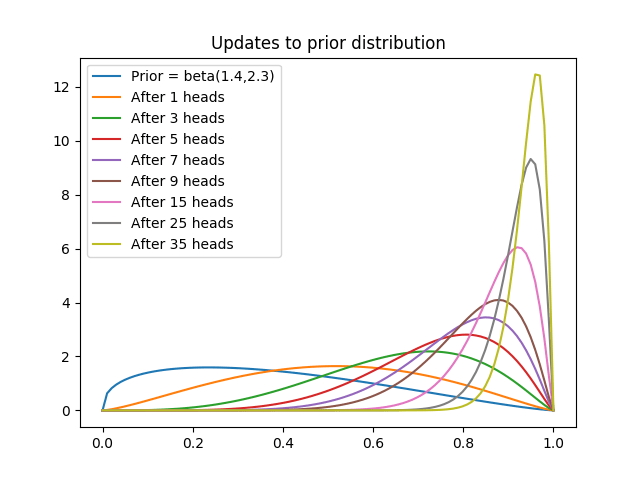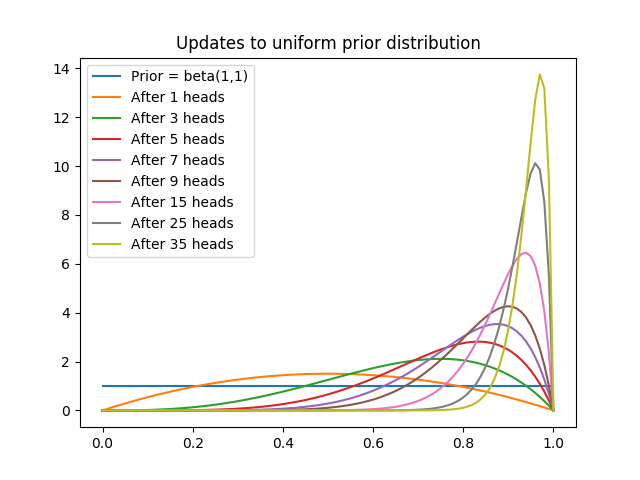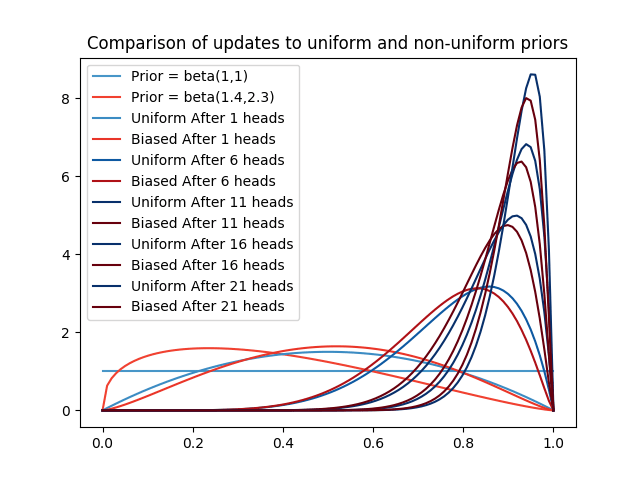# Parameter Estimation

…the statistician knows…that in nature there never was a normal distribution, there never was a straight line, yet with normal and linear assumptions, known to be false, he can often derive results which match, to a useful approximation, those found in the real world. – George Box (JASA, 1976, Vol. 71, 791-799)

## Parameter estimation

Suppose that we are given some data $D=(x_1,\cdots, x_n)\in T^n$. Our task is to find a parametric probability distribution $m(\hat{\theta})$ on $T$ such that if we were to independently sample from it $n$-times, we would could reasonably1 expect to obtain $D$. We first make some choice2 of possible distributions $m(-)$, which leaves us with the task of identifying the $\hat{\theta}$.

### Likelihood approach

One approach is the maximal likelihood estimate or MLE. For this we set $$\hat{\theta}=\theta_{MLE} = \mathrm{argmax}_\theta p(D | \theta).$$ That is we choose $\theta$ so that the probability density of $D$ from the given model is maximized.

There are a few comments that we should make here:

1. There may not be a maximum for a general distribution. Indeed the models typically considered for logistic regression do not have a maximum.
2. If there is a maximum, it may not be unique.
3. This is an optimization problem and when this problem is not convex, we may not have any method that is guaranteed to identify find a maximum even when it exists.

We do not actually address these problems, instead we just say that optimization techniques will give us a parameter value that is “good enough”.

#### Example

Suppose that $D=(x_1,\cdots, x_n)\in \Bbb R^n$ and we want to find a normal distribution $N(\mu, \sigma^2)$ which models the data under the assumption that these were independent samples.

First we construct the MLE of $\mu$:

\begin{align}

\end{align}

We differentiate the last expression with respect to $\mu$ and set this to 0 and obtain
\begin{align}
-\sum_{i=1}^n (x_i -\mu)&=0 \\
\mu_{MLE} &= \frac{\sum_{i=1}^n x_i}{n}.
\end{align}
Taking second derivatives shows that this is in fact a minimum. In other words, the MLE of $\mu$ is the sample mean.

#### Exercise

Show that MLE of $\sigma^2$ is the (biased) sample variance. In other words, $$\sigma_{MLE}^2 = \frac{\sum_{i=1}^n (x_i-\mu_{MLE})^2}{n}.$$

### Frequentist evaluation

The frequentist approach gives us a method to evaluate the above estimates of the parameters. Suppose that our data is drawn from a true distribution $m(\hat{\theta})$ and set $\overline{\theta} = E(\theta_{MLE}(D))$, where the expectation is given by integrating over $D$ given the “true” model. Define the bias of $\theta_{MLE}(-)$ by $$\mathrm{bias}(\theta_{MLE})=\overline{\theta}-\hat{\theta}.$$

Let $\mu_S(D)$ be the sample mean of data drawn from some distribution $m$ with finite mean $\widehat{\mu}$. Then we see
\begin{align}
E(\mu_S) & = E\left(\frac{\sum_{i=1}^n X_i}{n}\right) \\
& = \frac{\sum_{i=1}^n E(X_i)}{n} \\
& = n\widehat{\mu}/n = \widehat{\mu}
\end{align}
so this is an unbiased estimate.

For a visualization of an unbiased point estimate from the frequentist point of view look here.

#### Exercise

1. Show that the expected value of the sample variance $\sigma^2_S$ of data drawn from some distribution $m$ with finite mean $\widehat{\mu}$ and finite variance $\widehat{\sigma^2}$ is $$E(\sigma^2_S)= \frac{n \widehat{\sigma^2}}{n-1}.$$
Show that it follows that the bias of $\sigma^2_S$ is $\widehat{\sigma^2}/(n-1)$.
2. Show that the unbiased sample variance $\sigma^2_U(X_1,\cdots,X_n) = \frac{\sum_{i=1}^n(X_i-\mu_S(X_1,\cdots X_n))^2}{n-1}$ is, in fact, an unbiased estimate.
3. Construct a symmetric 95% confidence interval (see here for some additional discussion) for our estimate $\mu_S$ of $\widehat{\mu}$ which is centered about $\mu_S$, when $n=10$, $\mu_S=15$ and $\sigma^2_U = 2$. Hint: the random variable $\frac{\mu_S-\widehat{\mu}}{(\sigma_U /\sqrt{n})}$ has the same distribution as the Student’s $t$-distribution whose inverse cdf values can be calculated by statistics packages.

Suppose that we have an estimator $\theta=\delta(D)$ of $\hat{\theta}$ and the expected value of the estimate is $E(\delta(D))=\overline{\theta}$. We obtain the following expression for our expected squared error
\begin{align}
E((\theta-\hat{\theta})^2) &= E(\left((\theta-\overline{\theta})-(\hat{\theta}-\overline{\theta})\right)^2) \\
&= E((\theta-\overline{\theta})^2)-E(\theta-\overline{\theta})\cdot 2(\hat{\theta}-\overline{\theta}) + (\hat{\theta}-\overline{\theta})^2 \\
&= \mathrm{var}(\theta)+\mathrm{bias}^2(\theta)
\end{align}

This equation indicates that while it may be nice to have an unbiased estimator (i.e., one that will, on average, give precisely the correct parameter), if this comes with the cost of creating an estimate that varies wildly with the data then we will still expect a large amount of error and indicates why we would like estimators with low-variance.

A typical example of this is seen in polynomial regression (here we are viewing the problem as trying to estimate $p(y|x)$). As we vary the degrees of the polynomial being used, we find that very large degree polynomials can fit the data well:

However, the fit for high degree polynomials varies highly depending on the choice of data points:

### The MAP Estimate

One Bayesian approach to parameter estimation is called the MAP estimate or maximum a posteriori estimate. Here we are given data $D$, which we want to say is modeled by a distribution $m(\theta)$ and we construct the MAP estimate of $\theta$ as
\begin{equation}

\end{equation}
In other words, we choose the mode of the posterior distribution. Note that if our prior $p(\theta)$ is constant independent of $\theta$, then the only part of the right hand side that depends on $\theta$ is $p(D|\theta)$ and the MAP estimate of $\theta$ is the same as the MLE of $\theta$.

#### Example

Suppose that we have a coin whose bias $\theta$ we would like to determine (so $\theta$ is the probability of the coin coming up heads). We flip the coin twice and obtain some data $D=(H,H)$. Under the assumption that the coin flips are independent we are trying to model a Bernoulli distribution. Now we calculate the conditional probability:
\begin{equation}
p(D|\theta) = \theta^2(1-\theta)^0
\end{equation}
and quickly see the MLE for $\theta$ (given that $\theta \in [0,1]$) is what we would expect: $\theta = 1,$ as mentioned in the hypothesis testing example of an overconfident estimator.

As already mentioned, the MLE and MAP estimate agree in the case of the uniform prior, but the Bayesian approach allows us to calculate $p(\theta | D)$, which gives us a measure of our confidence in this estimate. In this case we see:
\begin{align}
p(\theta | D) &= \frac{p(D|\theta)p(\theta)}{\int_{\theta’} p(D|\theta’)p(\theta’)d\theta’} \\
&= \frac{\theta^2}{\int_{\theta’} (\theta’)^2d\theta’} \\
&= \frac{\theta^2}{1/3} \\
&= 3\theta^2.
\end{align}

If we want to find a 99.9% credible interval for the estimate $\theta_*\in [a,1]$, then we just integrate
\begin{align}
P(\theta \in [a,1] | D) &=\int_{\theta =a}^1 p(\theta | D)d\theta \\
&=\int_{\theta =a}^1 3\theta^2 d\theta \\
&=1-a^3.
\end{align}
Setting this to be equal to $1-10^{-3}$ we see that $a=0.1$. This interval is much more conservative than the confidence interval obtained by frequentist methods.

Now what if we do not have a uniform prior. Instead we suppose we have some prior information3 that makes us believe that the most likely value of $\theta$ is actually 0.25 and that it has variance 0.05. How do we incorporate this information into a prior? Well the mode and the variance are not sufficient to determine a distribution over the unit interval, so let’s assume that $p(\theta)$ has some convenient form that fits these values.

It would simplify matters if $p(\theta)$ had the same form as $p(D|\theta)=\theta^{|H|}(1-\theta)^{|T|}$. So, supposing $p(\theta)=C\theta^a(1-\theta)^b$ for some constant $C$ (i.e., $p(\theta)\propto \theta^a (1-\theta)^b$), we would see that
\begin{align}
p(\theta | D) &\propto p(D|\theta)p(\theta) \\
&\propto \theta^{|H|}(1-\theta)^{|T|} \theta^a(1-\theta)^b\\
& = \theta^{|H|+a}(1-\theta^{|T|+b}).
\end{align}
This has the same form as the prior! Such a prior is called a conjugate prior to the given likelihood function. Having such a prior is super convenient for computation.

In this case, the conjugate prior to the Bernoulli distribution is the Beta distribution which has the form $p(\theta | \alpha, \beta)\propto \theta^{\alpha-1}(1-\theta)^{\beta-1}$. One can calculate the mode and variance of this distribution (or just look it up) and get
\begin{align}
\textrm{mode} &= \frac{\alpha -1}{\alpha+\beta-2} \\
\sigma^2 &= \frac{\alpha \beta}{(\alpha+\beta)^2(\alpha+\beta+1)}
\end{align}
Plugging this into Wolfram Alpha gives us some approximate parameter values: $\alpha \approx 1.4$ and $\beta \approx 2.3$.

Using this prior, the new posterior is
$$p(\theta | {HH})\propto \theta^{2.4}(1-\theta)^{1.3}.$$ We can form the MAP estimate by taking the $\log$ of this expression (which is fine away from the points $\theta = 0, 1$, which we know can not yield the maximum), differentiating with respect to $\theta$, setting this to 0, and finally checking that the second derivative at this point is positive.

#### Exercise

Show the MAP estimate for this problem is $2.4/3.7\approx 0.65$ by calculating the mode of the $\beta$ density function.

We see that our estimate of $\theta$ shifts drastically as we obtain (even just a little bit) more information. Now lets see how the posterior changes as we see even longer strings of heads:From the image we see that as posterior gradually becomes highly confident that the the true value of $\theta$ is very large and assigns very small probability densities to small values of $\theta.$

To see how much our choice of prior affects the posterior in the presence of the same data, we can look at the analogous chart starting from a uniform prior, i.e., when the $\beta$ parameters are $\alpha=\beta=1$.Or we can look at a side by side comparison:From looking at the graphs, we can see that while the two priors are very different (e.g., the non-uniform prior assigns very small densities to large values of $\theta$), the posteriors rapidly become close to each other. How the posterior depends on the prior[/caption]Since they never agree, our methods still depend on our choice of prior, but we can also see that the both methods are approaching the same estimate.

To finally drive this point home, let’s consider a severely biased prior $\beta(1.01,10.0)$, whose mode puts the probability of heads at approximately 0 and with near 0 variance in the binomial model. Then we can see how the posterior changes with additional evidence:

The severely biased prior is much slower to approach the same consensus as the other priors.

#### Exercise

The severely biased prior is very close to what we would obtain as a posterior from a uniform prior after witnessing 9 tails in a row. If we were to witness 9 tails in a row followed by 20 heads in a row, how would you change your modeling assumptions to better reflect the data?

#### Further point estimates

As discussed above, one way to estimate a parameter is the MAP estimate which is just the mode of the posterior distribution $p(\theta | D)$. This is a very common approach to parameter estimation because it transforms the task into an optimization problem and we have a number of tools for such tasks.

However, the mode of a distribution can be far from a typical point. The mode of a distribution is also not invariant under reparametrization. One alternative is to select the mean or expected value of $\theta$ from the posterior distribution. Another alternative is to take the median of the posterior distribution.

1. I know this is not a very precise statement, but I want to be flexible at this point.
2. How we make this choice is the model selection problem which will be discussed later.
3. I apologize that this example is so contrived.